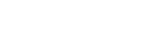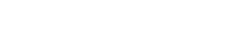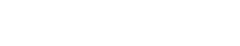## Questions

1. If tan xº = 12/5 and x is a reflex angle, find the value of 5sinx + cos x without using a calculator or mathematical tables
2. Find θ given that 2 cos 3θ -1 = 0 for 0º ≤ θ ≤ 360º
3. Without a mathematical table or a calculator, simplify:giving your answer in rationalized surd form.
4. Express in surds form and rationalize the denominator.5. Simplify the following without using tables;
Tan 45 + cos 45sin 60
6. Simplify the following surds in the form of a + √b c where a, b, and c are constants7. John cycles from shopping centre A on a bearing of 120º for 5 km to shopping centre B. He then cycles on a bearing of 200º for 7 km to the shopping centre C. Calculate to 1 decimal place.
1. The direct distance from A to C.
2. The bearing of A from C.
3. Bearing of B from C.

1.5 sin x + cos x
= 5 (12/13) − 5/13
= 60/135/13 = 55/13
= 12/13

2.3.4.5.6.1. b² = a² + c² – 2ac cos B
b² = 7² + 5² – 2.5.. 7 cos 100
= 74 – 70(-0.173648)
= 74 + 12.15537
b² = 86.15537
b = 9.28199
AC = 9.3 km
2. 9.3/sin100 = 5/sinθ
Sin θ = 5sin100/9.3 = 0.529466
θ = 31.9694
θ ≃ 32º
32 – 20 = 12º
Bearing = 360º - 12º = 348º
3. 020º

#### Download Trigonometry 2 Questions and Answers - Form 3 Topical Mathematics.

• ✔ To read offline at any time.
• ✔ To Print at your convenience
• ✔ Share Easily with Friends / Students

Read 9787 times Last modified on Wednesday, 10 February 2021 07:01
.
Subscribe now

access all the content at an affordable rate
or
Buy any individual paper or notes as a pdf via MPESA
and get it sent to you via WhatsApp